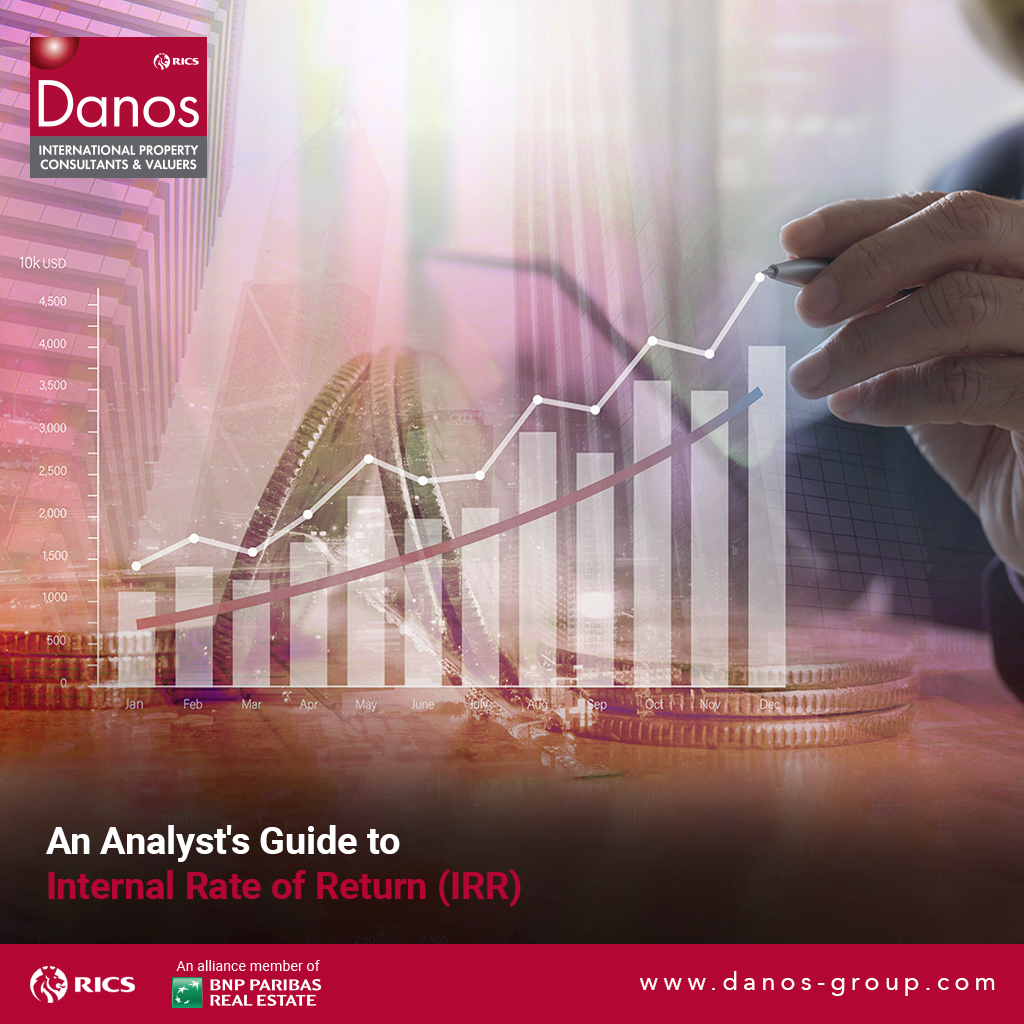# An Analyst’s Guide to Internal Rate of Return (IRR)## What is the Internal Rate of Return (IRR)?

The Internal Rate of Return (IRR) is the discount rate that makes the net present value (NPV) of a project zero. In other words, it is the expected compound annual rate of return that will be earned on a project or investment. In the example below, an initial investment of \$50 has a 22% IRR. That is equal to earning a 22% compound annual growth rate.